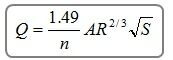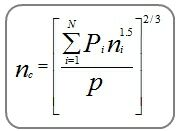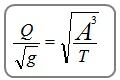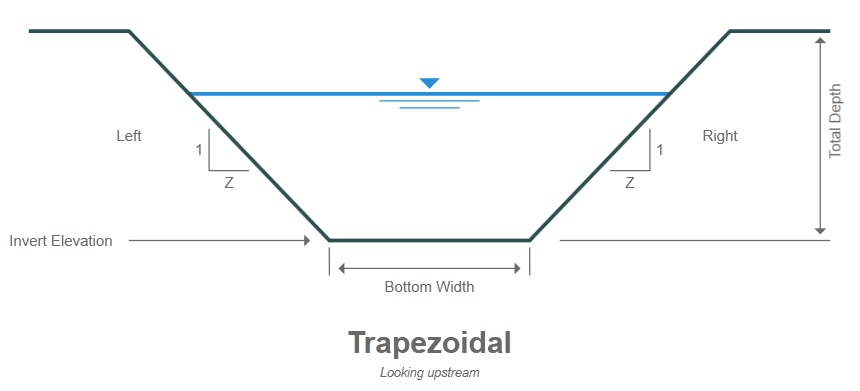1. Home
2. Computational Methods
3. Open Channels & Pipes

# Open Channels & Pipes

Studio Express computes various parameters for a variety of channel and pipe shapes. In all cases, steady flow is assumed and thus normal depth is assumed. Manning’s equation is used exclusively. When a known Q is specified it solves for the depth using an iterative procedure.

Manning’s EquationWhere:

Q = flow rate in cfs (cms)
n = roughness coefficient
A = cross-sectional area in sqft (sqm)
R = hydraulic radius = A / WP
S = channel slope in ft/ft (m/m)
Km = 1.49 (1.0)

Composite Manning’s n
With user-defined sections that have varying n values, Studio Express uses the following Horton-Einstein equation to first compute a composite roughness coefficient. Then it employs Manning’s equation as described above.Where:
nc = composite n-value
Pi = wetted perimeter of subdivision i
ni = n-value for subdivision i
p = total wetted perimeter of channel

Critical Depth
Yc, or critical depth is computed using the following equation along with an iterative procedure:Where:

Q = flow rate
A = cross-sectional area
T = top width